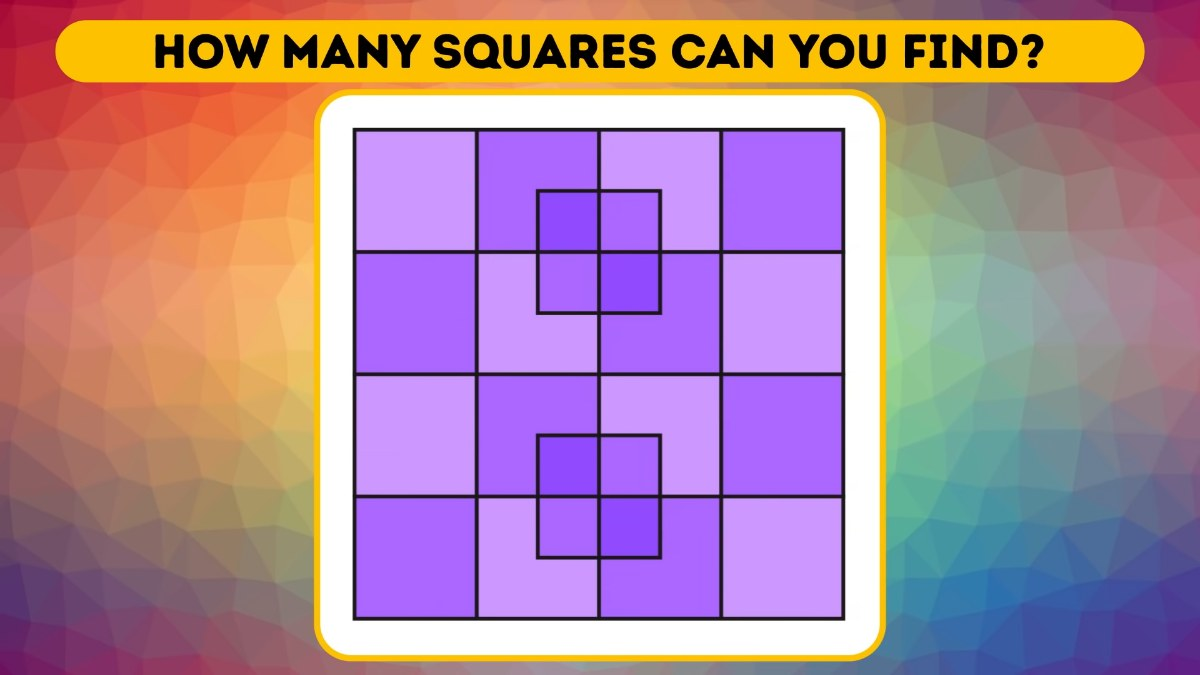# Math Riddle: Can You Count The Number Of Squares In This Picture?

This math riddle is for kids. Test your quantitative skills by counting how many squares are there in the picture.Math Riddle: Can You Count The Number Of Squares In This Picture?

When we hear the word "Math," we start imagining all the complicated problems involving numbers and alphabets, and other numerical expressions.

Whether you hate it or love it, having at least basic mathematical skills is necessary to advance your academic, professional, and personal lives.

This is why we are here with another math riddle and this time it is for kids. But, this math riddle can also be solved by adults.

So, let's begin.

Math Riddles With Answers: Can You Solve These Two Basic Math Problems?

## Can You Count The Number Of Squares In This Math Riddle?

Let's take a look at the picture of the square below.

Source: Bright Side

How many squares can you count in this picture?

But first, let's define what a square is. ⬜

A square is a quadrilateral with equal straight sides and four right angles.

Try And Solve This Math Riddle That Has Stumped Even Engineers.

Now, back to our math riddle.

How many squares will you count?

All the best!

The answer is provided below, but make sure you solve it by yourself first, without scrolling straight to the answer.

Math Riddle: Even Math Teachers Have Failed To Solve This Viral Stolen Bill Problem. Can You?

Math Riddle: Can You Solve This Children's Math Problem That Has Puzzled Even Adults?

In this math riddle, you had to count the total number of squares. We are revealing the correct answer now so that you can check whether you guessed correctly or not.

Answer. There are 40 squares in total.

Did you count the correct number of squares? Or did you guess wrong?

We hope you liked this math riddle.

Math Riddle For Exams: 5 Minute-Problems On Time, Speed, And Distance.

Get the latest General Knowledge and Current Affairs from all over India and world for all competitive exams.
खेलें हर किस्म के रोमांच से भरपूर गेम्स सिर्फ़ जागरण प्ले पर